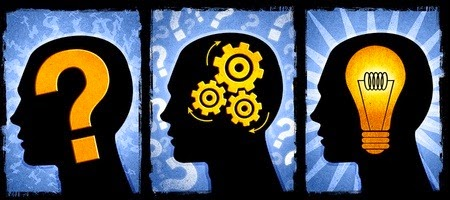# Reasoning Practice Test for SBI Bank Exams

Hi friends,  I am Shravan. Here I am sharing Reasoning Practice Questions for upcoming SBI Bank PO and Clerical exams. Happy Reading :)

Directions (Q. No. 1 - 5) : Each of the questions below consists of a question and two statements marked I and II given below it.

You have to decide whether the data provided in the statements are sufficient to answer the question.1. If the data in statement I alone are sufficient to answer the question, while the data in statement II alone are not sufficient to answer the question.
2.  If the data in statements I alone are not sufficient to answer the question, while the data in statement II alone are sufficient to answer the question.
3. If the data either in statement I or in statement II alone are sufficient to answer the question.
4. If the data even in both the statements I and II together are not sufficient to answer the quest-ion.
5. If the data in both statements I and II together are needed to answer the question.
1.  What is Virat's rank in a class of 50 students?
I. Suraj, whose rank is 17 th in the class, is ahead of Ghana by 8 ranks. There are 7 persons between Ghana and Virat.
II. Sivramesh is 20 ranks ahead of Virat and Malala is 6 ranks behind Virat. Sangita stands exactly in the middle of Malala and Sivramesh.

2. How many daughters does Y have?
I. E and Q are the only daughters of Z.
II. D is brother of Q and son of Y.

3. What does "De" stands for in the code language?
I. "Pa Ni Nok De" means "better late than never" and "Ri Nok De Pam" means "never be so late" in that code language.
II. "Mi De Mo Nam" means "being late is lazy" and "De Mok Sac Li" means "Late moves spoil plans" in that code language.

4. Who amongst P, Q, R, S, T and U, who are at different weights, is the heaviest?
I. P is lighter than only R.
II. Only R is heavier than P.

5. Towards which direction is A from Z?
I. A is exactly to the south east of M.
II. Z is exactly to the south of M.

Directions (Q. No. 6-10)
: Observe the statements carefully and choose the option by finding out the validity of the given conclusions:
6. Statements : All Rats are Cats. Some Cats are planets. All planets are Tiles.
Conclusions : I. Some Tiles are Rats.
II. No Rat is a Tile.
III. All Tiles being planets is a possibility
1) Only I, II and III follow
2) Only II and III follow
3) Only III with Either I or II follows
4) I and III follow
5) None of these

7. Statements : All Fans are bats. All bats are rats. All rats are cots.
Conclusions : I. Some cots are not bats
II. All cots are bats
III. All those rats which are fans must be bats
1) Only II and III follow
2) Only I and III follow
3) Either I or II and III follow
4) All follow
5) None of these

8. Statements : All curtains are rods. Some rods are sheets. Some sheets are pillows.
Conclusions : I. Some pillows are rods.
II. Some rods are curtains.
III. Some pillows which are sheets need not necessarily be rods.
1) Only I and III follow
2) Only II and III follow
3) Either I or III and II follows
4) All follow
5) None of these

9. Statements : No wall is roof. All pens are walls. All doors are roofs.
Conclusions : I. Some doors are walls.
II. No roof is wall
III. No any Pen is door
1) Only I and II follow
2) Only II and III follow
3) Either I or II and III follows
4) All follow
5) None of these

10. Statements :
All switches are plugs. Some plugs are bulbs. All bulbs are sockets.
Conclusions : I. At least Some sockets are plugs
II. Some plugs are switches.
III. All sockets can never be switches
1) Only I and II follow
2) All follow
3) Either I or III and II follows
4) None follows
5) None of these

Directions (Q. No. 11 - 15) : Study the information carefully and answer the following questions:
In a certain code language-
a) "GOOD WORK EARNS MONEY" is written as "kat fat ho gat"
b) "PRODUCTIVE AND EXCELLENT WORK" is written as, 'mat ta pat kat'
c) "MONEY IS DIRECT OUTPUT" is written as, "zi lat ne ki"
d) "WORK PRODUCES EXCELLENT RESULTS" is written as, "zat mat kat da"

11. What is the code for "WORK" in the given code language?
1) gat
2) ho
3) pat
4) kat
5) da

12. What is the code for "EXCELLENT" in the given code ?
1) kat
2) da
3) mat
4) gat
5) zi

13. What is the code for "MONEY" in the given code language?
1) lat
2) ho
3) kat
4) zi
5) da

14. What is the code for "RESULTS" in the given code language?
1) zat
2) da
3) either 1 or 2
4) mat
5) da

15. What is the code for "PRODUCTIVE" in the given code?
1) mat
2) pat
3) kat
4) ta
5) cannot be determined

Check Solutions for above Reasoning problems from here